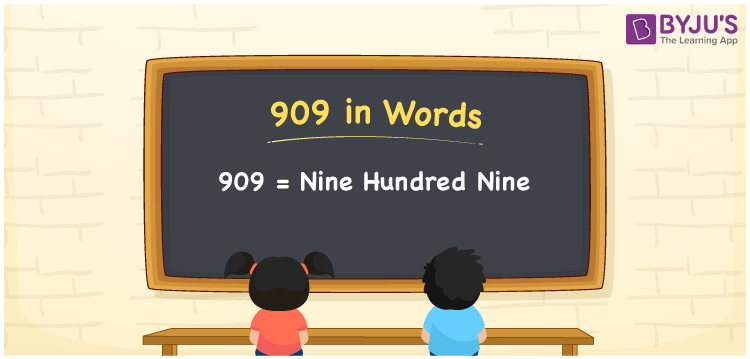# 909 in words

909 in words is written as Nine Hundred and Nine. 909 represents the count or value. The article on Counting Numbers can give you an idea about count or counting. The number 909 is a 3 digit number that is used in expressions related to money, days, distance, length, weight and so on. Let us consider an example for 909. “Reena gave me Nine rupees to me, so the total amount from my piggy bank is Nine Hundred and Nine rupees”.

 909 in words Nine Hundred and Nine Nine Hundred and Nine in Numbers 909

## 909 in English Words## How to Write 909 in Words?

We can convert 909 to words using a place value chart. The number 909 has 3 digits, so let’s make a chart that shows the place value up to 3 digits.

 Hundreds Tens Ones 9 0 9

Thus, we can write the expanded form as:

9 × Hundred + 0 × Ten + 9 × One

= 9 × 100 + 0 × 10 + 9 × 1

= 909

= Nine Hundred and Nine.

909 is the natural number that is succeeded by 908 and preceded by 910.

909 in words – Nine Hundred and Nine.

Is 909 an odd number? – Yes.

Is 909 an even number? – No.

Is 909 a perfect square number? – No.

Is 909 a perfect cube number? – No.

Is 909 a prime number? – No.

Is 909 a composite number? – Yes.

## Solved Example

1. Write the number 909 in expanded form

Solution: 9 × 100 + 0 × 10 + 9 × 1

We can write 909 = 900 + 00 + 9

= 9 × 100 + 0 × 10 + 9 × 1.

## Frequently Asked Questions on 909 in words

### How to write the number 909 in words?

909 in words is written as Nine Hundred and Nine.

### Is 909 divisible by 9?

Yes. 909 is divisible by 9.

### Is 909 a prime number?

No. 909 is not a prime number.